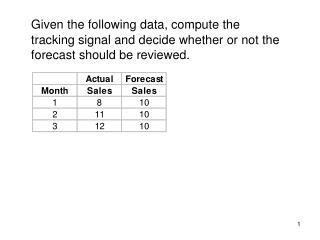# Forecast Error = Actual – Forecast = 8 -10 = -2 - PowerPoint PPT PresentationDownload PresentationForecast Error = Actual – Forecast = 8 -10 = -2

Forecast Error = Actual – Forecast = 8 -10 = -2Download Presentation## Forecast Error = Actual – Forecast = 8 -10 = -2

- - - - - - - - - - - - - - - - - - - - - - - - - - - E N D - - - - - - - - - - - - - - - - - - - - - - - - - - -
##### Presentation Transcript

1. Given the following data, compute the tracking signal and decide whether or not the forecast should be reviewed.

2. Tracking signal is computed as the running sum of forecast error (RSFE) divided by MAD. We compute RSFE by summing up the forecast errors over time. Forecast errors for January is the difference between its actual and forecast sales. RSFE for January is equal to the cumulative forecast errors. RSFE = -2 Forecast Error = Actual – Forecast = 8 -10 = -2

3. Forecast errors for February is the difference between its actual and forecast sales. RSFE for February is equal to the cumulative forecast errors of January and February. RSFE = -2 + 1 = -1 Forecast Error = Actual – Forecast = 11 -10 = 1

4. Forecast errors for March is the difference between its actual and forecast sales. RSFE for March is equal to the cumulative forecast errors of January, February and March. RSFE = -1 + 2 = 1 Forecast Error = Actual – Forecast = 12 -10 = 2

5. Forecast errors for April is the difference between its actual and forecast sales. RSFE for April is equal to the cumulative forecast errors of January, February, March and April. Forecast Error = Actual – Forecast = 14 -10 = 4 RSFE = 1 + 4 = 5

6. MAD for January is computed by averaging the absolute errors over time. Tracking signal for January is computed by dividing its RSFE by MAD. TS = RSFE/MAD = -2/2 = -1 Absolute Error = Absolute (Actual – Forecast) = Absolute(8 -10) = 2 MAD = 2

7. MAD for February is computed by averaging the absolute errors of January and February. Tracking signal for February is computed by dividing its RSFE by MAD. TS = RSFE/MAD = -1/1.5 = -0.67 Absolute Error = Absolute (Actual – Forecast) = Absolute(11 -10) = 1 MAD = (2+1)/2 = 1.5

8. MAD for March is computed by averaging the absolute errors of January, February and March. Tracking signal for March is computed by dividing its RSFE by MAD. TS = RSFE/MAD = 1/1.67 = 0.6 Absolute Error = Absolute (Actual – Forecast) = Absolute(12 -10) = 2 MAD = (2+1+2)/3 = 1.67

9. MAD for April is computed by averaging the absolute errors of January, February, March and April. Tracking signal for April is computed by dividing its RSFE by MAD. Absolute Error = Absolute (Actual – Forecast) = Absolute(14 -10) = 4 TS = RSFE/MAD = 5/2.25 = 2.22 MAD = (2+1+2+4)/4 = 2.25

10. Since the tracking signals for months January to April are within +/- 4, the forecast needs not be reviewed.